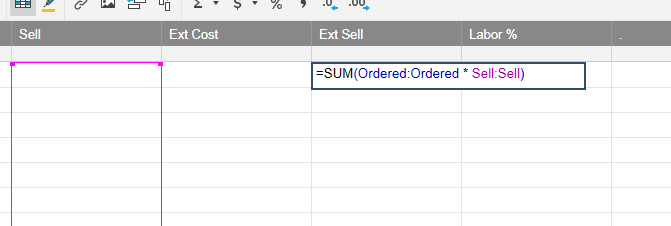# Multiply 2 Columns and display the result

Hello

I have an "Ordered" column and "Sell" column. I want to multiply the value in Ordered by Sell to generate an Ext Sell value in a third column.

I have tried the following formula in the Ext Sell column but I get #invalid operation

=SUM(Ordered:Ordered * Sell:Sell)

Where have I gone wrong?

Thank you• Hi MRCNathan,

If you are trying to multiply Ordered and Sell on each row and display the result in Exit Sell:

=Ordered1 * Sell1

Then drag the formula down the column as far as you require via the little square in the bottom right of the first Exit Sell cell.

I'd then suggest locking the column by right clicking on the Exit Sell column title and selecting Lock Column.

From then on in, when you add new data into the next blank row, the Exit Sell column will copy the formula from the last populated cell above and you will stilll see a result in Exit Cell.

If you are trying to multiply everything in Ordered by everything in Sell, place this formula in the Exit Sell row:

=SUM(Ordered:Ordered)*SUM(Sell:Sell)

Kind regards,

Chris McKay